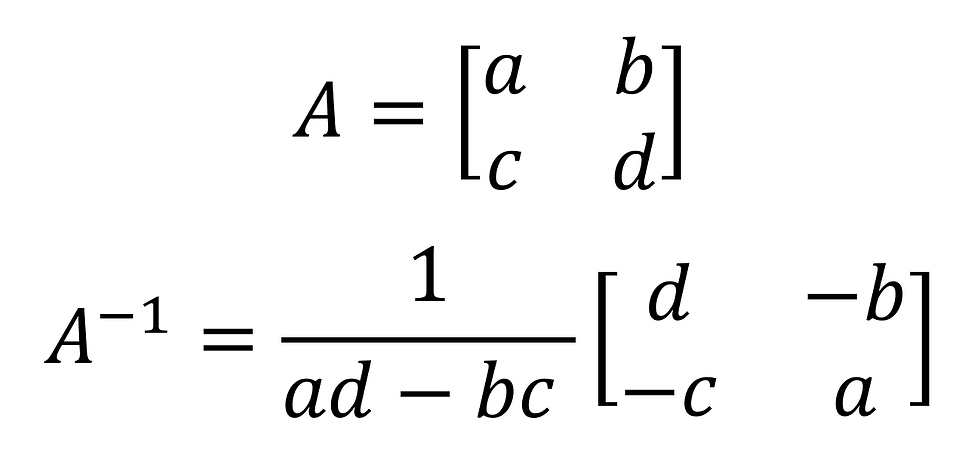cum
top of page
Search

# CSEC Mathematics: Matrices- Determinants and Inverse

Finding the Determinant of a 2 x 2 Matrix

The determinant of a matrix is a very important value that will help us later on when we are finding the inverse of a matrix, and when solving systems of linear equations.

The determinant of a matrix C is shown using det C or |C|.

To find the determinant of a 2 x 2 matrix:As you can see in the image, you simply add the products of the diagonals.

Example:Therefore, the determinant of A is -49.

Finding the Inverse of a 2 x 2 Non-Singular Matrix

The inverse of a matrix can only be found if that matrix is non-singular and square. That is, if the determinant of the matrix is not equal to zero and the number of rows is equal to the number of columns.

This is how you find the inverse of a non-singular 2x2 matrix:In the first part of the answer, we find the reciprocal of the determinant. Then, we switch the places of the a and d terms and make the b and c terms negative (this is a shortcut to finding a term called the adjoint). By multiplying these two terms together, we find our inverse.

For example:However, this only works for 2 x 2 matrices. There is another method that works for all matrices (CSEC will only give you problems involving the determinant for 2 x 2 matrices though, so it is unnecessary to learn this other method. You can read up on it here if you want to, though).